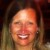# Sample Size Estimation

Six Sigma – iSixSigma Forums General Forums Tools & Templates Sample Size Estimation

Viewing 5 posts - 1 through 5 (of 5 total)
• Author
Posts
• #248533irfan.ahanger01
Participant

I have 100 batches of process data and I would like to estimate a sample size that is a representative of the entire population (covers at least 80% of the population). Please suggest different ways of calculating the sample size.

0
#248536Katie Barry
Participant

@irfan.ahanger01 What do you think you should do? Our audience is extremely helpful but they’re much more likely to respond if you share your thoughts on your proposed approach first.

If you haven’t already, you may want to check out our sampling section of content: https://www.isixsigma.com/tools-templates/sampling-data/

1
#248543Robert Butler
Participant

As written, the answer to your question is to sample 80% of the data – there’s no estimation involved.

Sample size questions focus on moments of a distribution (things like means, standard deviations, etc.)of a sample and are phrased in the following manner: How many samples do I need to take in order to be certain that the mean/standard deviation/percent defective/percent response/percent change/etc. of the sample is not significantly different from a target?  Where the target can be things like – customer specified  means or standard deviations, gold standard targets, moment measures of an earlier sample from the process before we made a change, etc.

So, if you are interested in sample sizes then your question needs to be recast – for example:

I have 100 batches of process data. How many samples will I need to take to be 80% certain that my sample mean is within plus/minus some range of a target mean?

1
#248555irfan.ahanger01
Participant

Thank you so much Mr. Robert.

Yes, you are absolutely right. I need to be X% certain that my sample mean is within plus/minus some range (i.e., measurement error, 1-3SD). Please suggest the way I can calculate the sample size that covers 80% of the population with 95% confidence (Tolerance)?

0
#248564Robert Butler
Participant

I think you are still misunderstanding the issue of sample size. Your statement “…covers 80% of the population with 95% confidence…” has no meaning.

Let’s pretend you have done all of the usual things you need to do with respect to visually inspecting the distribution of those 100 samples (histogram, data on a normal probability plot, time plot of data, etc.) and what you have observed is a uni-modal distribution which is “acceptably” symmetric (this being a judgement call) and whose time plot is “acceptably” random (no obvious trending over time).

Given this the next step would be to compute the sample mean of those 100 samples and its associated standard deviation.

Armed with this information you can address any of the following questions:

1. How small a sample would I need to compare the mean of a new sample to the mean of the existing sample and be certain with a probability of 95% that the mean of the new sample was not significantly different from the mean of the 100 sample baseline?

2. How small a sample would I need to compare the standard deviation of a new sample to the standard deviation of the existing sample and be certain with a probability of 95% that the standard deviation of the new sample was not significantly different from the mean of the 100 sample baseline?

3. Given the 100 sample mean and the 100 sample standard deviation what kind of a spread around the sample mean would encompass 95% of the data – or, in your case, what kind of a spread around the sample mean would encompass 80% of the data where the data is:

a. existing/future individual samples
b. the means of samples of size X where X is is the count of a new sample whose total is something less than 100
c. the means of future samples of the same size as my original (100 samples)

Given what you have posted my guess (and I could be wrong about this) is question 3a is the one you want answered.

If you want to know the expected range of individual measurements around the mean of the results from your 100 samples then the equation would be:

#1 Range = sample mean of the 100  +- (1.987*sample standard deviation/sqrt(n))  where n is the sample size. Since you are interested in the estimate of the range for a single sample n = 1.  The 1.987 is an extrapolation for the fractional points of a t Distribution where for 95% the value for 60 samples is 2.00 and the value for 120 samples is 1.98.

So, the estimate of the range of single values for 80% of the population would be:

#2 Range = sample mean of the 100  +- (1.291*sample standard deviation/sqrt(n))  where, again, n = 1 and 1.291 is the extrapolation for the fractional points of a t Distribution where, for 80%,  the value for 60 samples is 1.296 and the value for 120 samples is 1.289.

Thus, if you took a new measurement from the same process and you wanted to be sure that single measurement could be viewed as coming from the central 80% of the distribution of your process output (as defined by you 100 sample check) you would examine it relative to the #2 Range – if it was inside those limits then you would have no reason to believe it was not a sample which was representative of the central 80% of your process output.

0
Viewing 5 posts - 1 through 5 (of 5 total)

You must be logged in to reply to this topic.